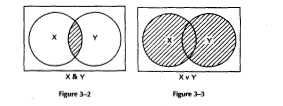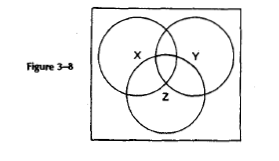# 3.1: Logical Equivalence

I introduced logic as the science of arguments. But before turning to arguments, we need to extend and practice our understanding of logic's. basic tools as I introduced them in chapter 1. For starters, let's look at the truth table for 'A', '~A', and the negation of the negation of 'A', namely, '~~A'

 A ~A ~~A t f t f t f

This truth table exhibits the special situation which I mentioned at the end of the last chapter: The truth value of '~~A' is always the same as that of 'A'. Logicians say that 'A' and '~~A' are Logically Equivalent.

As we will see in a moment, much more complicated sentences can be logically equivalent to each other. To get dear on what this means, let us review some of the things that truth tables do for us. Suppose we are looking at a compound sentence, perhaps a very complicated one which uses many sentence letters. When we write out the truth table for such a sentence, we write out all the possible cases, that is, all the possible assignments of truth values to sentence letters in all possible combinations. In each one of these possible cases our original sentence has the truth value t or the truth value f.

Now suppose that we look at a second sentence which uses the same sentence letters, or perhaps only some of the sentence letters that the first sentence uses, and no new sentence letters. We say that the two sentences are logically equivalent if in each possible case, that is, for each line of the truth table, they have the same truth value.

Two sentences of sentence logic are Logically Equivalent if and only if in each possible case (for each assignment of truth values to sentence letters) the two sentences have the same truth value.

What we said about the double negation of 'A' naturally holds quite generally:

The Law of Double Negation (DN): For any sentence X, X and ~~X are logically equivalent.

Here are two more laws of logical equivalence:

De Morgan's Laws (DM): For any sentences X and Y, ~(X&Y) is logically equivalent to ~Xv~Y. And ~(XvY) is logically equivalent to ~X&~Y.

Thus 'Adam is not both ugly and dumb.' is logically equivalent to 'Either Adam is not ugly or Adam is not dumb.' And 'Adam is not either ugly or dumb.' is logically equivalent to 'Adam is not ugly and Adam is not dumb.' You should check these laws with truth tables. But I also want to show you a second, informal way of checking them which allows you to "see" the laws. This method uses something called Van Diagram.

A Venn diagram begins with a box. You are to think of each point inside the box as a possible case in which a sentence might be true or false. That is, think of each point as an assignment of truth values to sentence letters, or as a line of a truth table. Next we draw a circle in the box and label it with the letter 'X', which is supposed to stand for some arbitrary sentence, atomic or compound. The idea is that each point inside the circle represents a possible case in which X is true, and each point outside the circle represents a possible case in which X is false.

Look at Figure 3-1. What area represents the sentence ~X? The area outside the circle, because these are the possible cases in which X is false.

Now let's consider how Venn diagrams work for compound sentences built up from two components, X and Y. Depending on what the sentences X and Y happen to be, both of them might be true, neither might be true, or either one but not the other might be true. Not to omit any ofthese eventualities, we must draw the circles representing X and Y as overlapping, as in Figure 3-2 and 3-3.The conjunction X&Y is true in just those cases represented by points that lie inside both the X and Y circles, that is, the shaded area in Figure 3-2. The disjunction XvY is true in just those cases represented by points that lie inside either the X or the Y circle (or both), that is, the shaded area in Figure 3-3.

Now we can use Venn diagrams to check De Morgan's laws. Consider first a negated conjunction. Look for the area, shown in Figure 3-4, which represents ~(X&Y). This is just the area outside the shaded lens in Figure 3-2.Let us compare this with the area which represents ~Xv~Y. We draw overlapping X and Y circles. Then we take the area outside the first circle (which represents ~X; see Figure 3-5), and we take the area outside the second (which represents ~Y; see Figure 3-6).

Finally, we put these two areas together to get the area representing the disjunction ~Xv~Y, as represented in Figure 3-7.Notice that the shaded area of Figure 3-7, representing ~Xv~Y, is the same as that of Figure 3-4, representing ~(X&Y). The fact that the same shaded area represents both ~Xv~Y and ~(X&Y) means that the two sentences are true in exactly the same cases and false in the same cases. In other words, they always have the same truth value. And that is just what we mean by two sentences being logically equivalent.

Now try to prove the other of De Morgan's laws for yourself using Venn diagrams.

Here are two more laws of logical equivalence:

The Dishibutive Laws: For any three sentences, X, Y, and Z, X&(YvZ) is logically equivalent to (X&Y)v(X&Z). And Xv(Y&Z) is logically equivalent to (XW&(XvZ).

For example, 'Adam is both bold and either clever or lucky.' comes to the same thing as 'Adam is either both bold and clever or both bold and lucky.' You should prove these two laws for yourself using Venn diagrams. To do so, you will need a diagram with three circles, one each representing X, Y, and Z. Again, to make sure that you omit no possible combination of truth values, you must draw these so that they all overlap, as in Figure 3-8.Fill in the areas to represent YvZ, and then indicate the area which represents the conjunction of this with X. In a separate diagram, first fill in the areas representing X&Y and X&Z, and then find the area corresponding to the disjunction of these. If the areas agree, you will have demonstrated logical equivalence. Do the second of the distributive laws similarly. Also, if you feel you need more practice with truth tables, prove these laws using truth tables.

Exercise

3-1. Prove the second of De Morgan's laws and the two distributive laws using Venn diagrams. Do this in the same way that I proved the first of De Morgan's laws in the text, by drawing a Venn diagram for each proof, labeling the circles in the diagram, and explaining in a few sentences how the alternate ways of getting the final area give the same result. Use more than one diagram if you find that helpful in explaining your proof.